### MEMO 2017 ekipno problem 8

Kvaliteta:
Avg: 0,0
Težina:
Avg: 6,0

For an integer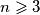$n \geqslant 3$ we define the sequence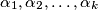$\alpha_1, \alpha_2, \ldots, \alpha_k$ as the sequence of exponents in the prime factorization of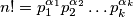$n! = p_1^{\alpha_1}p_2^{\alpha_2} \ldots p_k^{\alpha_k}$, where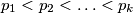$p_1 < p_2 < \ldots < p_k$ are primes. Determine all integers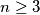$n \geq 3$ for which$\alpha_1, \alpha_2, \ldots, \alpha_k$ is a geometric progression.

Izvor: Srednjoeuropska matematička olimpijada 2017, ekipno natjecanje, problem 8